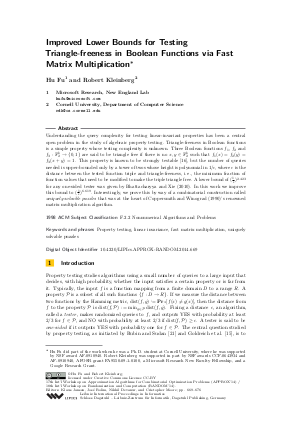Document# Improved Lower Bounds for Testing Triangle-freeness in Boolean Functions via Fast Matrix Multiplication

### Authors Hu Fu, Robert Kleinberg## File

LIPIcs.APPROX-RANDOM.2014.669.pdf
• Filesize: 456 kB
• 8 pages

## Cite As

Hu Fu and Robert Kleinberg. Improved Lower Bounds for Testing Triangle-freeness in Boolean Functions via Fast Matrix Multiplication. In Approximation, Randomization, and Combinatorial Optimization. Algorithms and Techniques (APPROX/RANDOM 2014). Leibniz International Proceedings in Informatics (LIPIcs), Volume 28, pp. 669-676, Schloss Dagstuhl - Leibniz-Zentrum für Informatik (2014)
https://doi.org/10.4230/LIPIcs.APPROX-RANDOM.2014.669

## Abstract

Understanding the query complexity for testing linear-invariant properties has been a central open problem in the study of algebraic property testing. Triangle-freeness in Boolean functions is a simple property whose testing complexity is unknown. Three Boolean functions f1, f2 and f3, mapping {0,1}^k to {0,1}, are said to be triangle free if there is no x, y in {0,1}^k such that f1(x) = f2(y) = f3(x + y) = 1. This property is known to be strongly testable (Green 2005), but the number of queries needed is upper-bounded only by a tower of twos whose height is polynomial in 1 / epsislon, where epsislon is the distance between the tested function triple and triangle-freeness, i.e., the minimum fraction of function values that need to be modified to make the triple triangle free. A lower bound of (1 / epsilon)^2.423 for any one-sided tester was given by Bhattacharyya and Xie (2010). In this work we improve this bound to (1 / epsilon)^6.619. Interestingly, we prove this by way of a combinatorial construction called uniquely solvable puzzles that was at the heart of Coppersmith and Winograd's renowned matrix multiplication algorithm.
##### Keywords
• Property testing
• linear invariance
• fast matrix multiplication
• uniquely solvable puzzles

## Metrics

• Access Statistics
• Total Accesses (updated on a weekly basis)
0